# Test: Coordinate Geometry - 2

## 25 Questions MCQ Test Mathematics (Maths) Class 9 | Test: Coordinate Geometry - 2

Description
Attempt Test: Coordinate Geometry - 2 | 25 questions in 25 minutes | Mock test for Class 9 preparation | Free important questions MCQ to study Mathematics (Maths) Class 9 for Class 9 Exam | Download free PDF with solutions
QUESTION: 1

Solution:
QUESTION: 2

Solution:
QUESTION: 3

### The point (3, 2) is at a distance of _______________ units from y-axis :

Solution:
QUESTION: 4

The point (–3, 2) belongs to Quadrant _______________ :

Solution:
QUESTION: 5

The point (2, –3) belongs to quadrant _______________ :

Solution:

The x value is POSITIVE which means that the point is to the right of the origin.

The y value is NEGATIVE which means that the point is below the origin.

The 4th quadrant is to the right and below the origin,(The bottom right quadrant)

All points in the 4th quadrant have the signs as (+,−)

QUESTION: 6

The point (3, 2) belongs to quadrant _______________ :

Solution:
QUESTION: 7

The point (–2, –3) belongs to Quadrant :

Solution:
QUESTION: 8

The point (–2, 0) lies on :

Solution:
QUESTION: 9

The point (0, –2) lies on :

Solution:
QUESTION: 10

The point (3, 0) lies on :

Solution:
QUESTION: 11

The point (0, 3) lies on :

Solution: This is because y is also called X=0 and x=0,y=3 so it will lie on positive y axis(+ve y axis)
QUESTION: 12

The distance between the points (–4, 7) and (1, –5) is :

Solution:

Use the distance formula to determine the distance between two points.

√{1-(-4)}2 +{(-5)-7}2

=√25+144

=√169

=13

QUESTION: 13

The distance of the point (–2, –2) from the origin is :

Solution: Solving it by Pythagoras theorem the perpendicular will be 2 and the base will also be 2 the distance will be the hypotenuse =>. h² = b² + p² h²= 2² + 2² h² = 4+4 h²= 8 h=√8 √8 can be written as √2 x √2 x √2 which is equal to 2√2units.
QUESTION: 14

If the distance between points (p, –5), (2, 7) is 13 units, then p is _____________ :

Solution:

Distance between two points (x1,y1) and (x2,y2) can be calculated using the formula −

Distance between the points (p,−5) and (2,7)=

(2−p)2  +(7−(−5)) 2 =13

(2-p)2 + 144 = 13

(2-p)2 + 144 = 169

(2-p)2  = 25

2-p = 5  or 2-p = -5

p = -3 or p = 7

QUESTION: 15

The points (a, a) (–a, a) and (– (√3) a, (√3)a) form the vertices of an :

Solution:
QUESTION: 16

Find the ratio in which the line joining the points (6, 4) and (1, –7) is divided by x-axis.

Solution: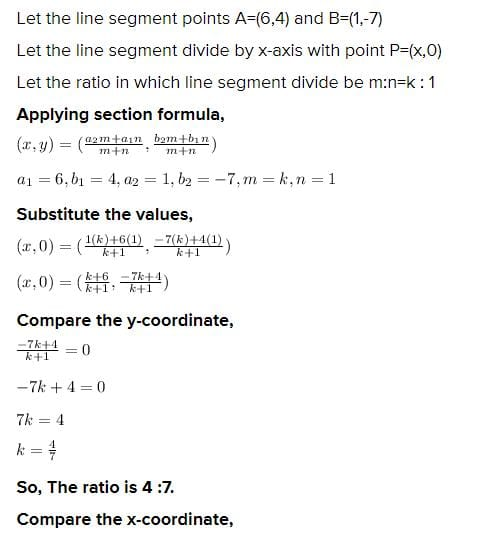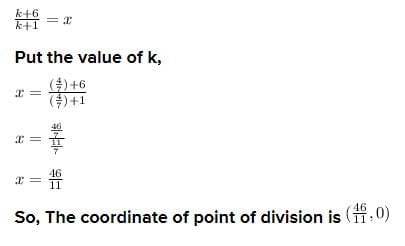QUESTION: 17

The points (2, –1), (3, 4), (–2, 3) and (–3, –2) taken in the order from the vertices of _________________:

Solution: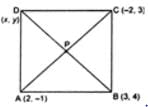In rhombus, all sides are equal and diagonals are not equal.

Distance between two points = [(x2−x1)2 + (y2−y1)2]1/2

AB =  [(3−2)2 + (4+1)2]1/2 =(26)1/2

BC = [(3+2)2 + (3−4)2]1/2 = (26)1/2

CD = [(-3+2)2 + (-2-3)2]1/2 = (26)1/2

DA = [(-3-2)2 + (-2+1)2]1/2 = (26)1/2

AC = [(2+2)2 + (4)2]1/2 = 4(2)1/2

BD = [(-3-3)2 + (4+2)2]1/2 = 6(2)1/2

AB=BC=CD=DA All sides are equal

AC and BD Diagonals are not equal

QUESTION: 18

The points (0, 0), (–2, 0) and (3, 0) _________________:

Solution:
QUESTION: 19

The point on Y-axis equidistant from (–3, 4) and (7, 6) is _________________:

Solution:
QUESTION: 20

The point on X-axis equidistant from (5, 4) and (–2, 3) is _________________:

Solution:
QUESTION: 21

The points on X-axis at a distance of 10 units from (11, –8) are :

Solution:

Let P(x, 0) be the point on the x-axis. Then as per the question we have

AP = 10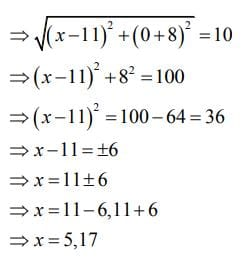Hence, the points on the x-axis are (5, 0) and (17,0) .

QUESTION: 22

The points on Y-axis at a distance of 13 units from point (–5, 7) are :

Solution:
Let  P(0,y) be the required point on y−axis.
Now, distance between the point P(0,y) and A(−5,7) is 13 units.
Now, PA = 13 units
⇒√(0+5)^2 + (y−7)^2 = 13
⇒√25 + y2 + 49 − 14y = 13
⇒√y^2 + 74 − 14y = 13
⇒y^2 − 14y + 74 = 169
⇒y^2 − 14y − 95 = 0
⇒y^2 − 19y + 5y − 95 = 0
⇒y(y−19) + 5(y−19) = 0
⇒(y+5)(y−19) = 0⇒y+5 = 0   or  y−19 = 0
⇒y = −5   or  y = 19
So, the required points are :(0,−5) and (0,19)
QUESTION: 23

The coordinates of the centre of a circle passing through (1, 2), (3, –4) and (5, –6) is _________________:

Solution:

Given three points (1,2),(3,−4)and(5,−6)

Let the coordinates o centre be O(h,k)

The distance from the centre to a point on the circumference is equal to radius.

∴ OA=OB=OC

From distance formula

OA2=OB2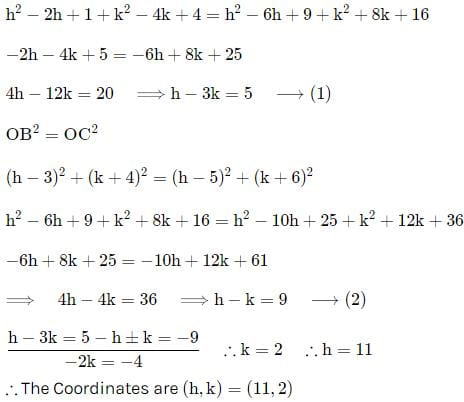QUESTION: 24

The points (–5, 6), (3, 0) and (9, 8) form the vertices of :

Solution:
QUESTION: 25

The points (4, 4), (3, 5) and (–1, –1) from the vertices of :

Solution:Use Code STAYHOME200 and get INR 200 additional OFF Use Coupon Code